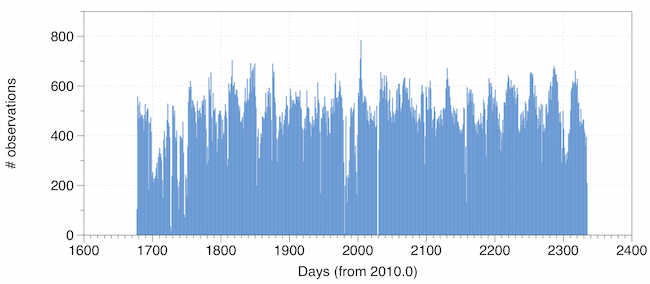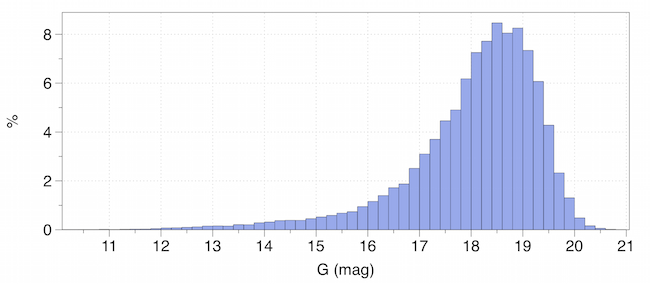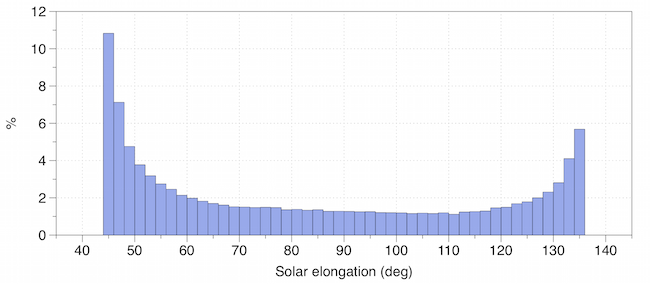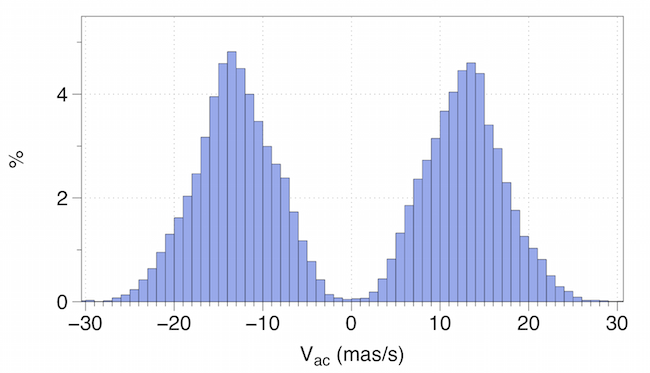# 4.2.2 Observed properties versus expected ones

Author(s): François Mignard, Paolo TangaFigure 4.11: Distribution of the expected number of transits for the 14 125 selected SSOs processed in the Gaia DR2.Figure 4.12: Distribution of the expected number of transits par day for the 14 125 selected SSOs processed in the Gaia DR2.Figure 4.13: Distribution of the G magnitude of the SSOs for the 318 290 transits selected in the Gaia DR2.

## Number of observations per object

An observation is defined here as a transit in one of the Gaia fields of view. The expected number of transits shown in Figure 4.11 is based on the list of 14 125 selected SSOs. It corresponds to the $318\,290$ passages found in the IDT data. This was the maximum number of transits that could have entered the processing chain. However, the actual number of transits in the final output is slightly smaller, since not every passage produced an acceptable astrometric solution for various reasons.Figure 4.14: Distribution of solar elongations of the 318 290 transits selected in the Gaia DR2.Figure 4.15: Distribution of the along-scan velocity of the 318 290 transits selected in the Gaia DR2. Restricted to the elongations <90 ∘ (left), and >90 ∘ (right).Figure 4.16: Distribution of the across-scan velocity of the 318 290 transits selected in the Gaia DR2. Restricted to the elongations <90 ∘ (left), and >90 ∘ (right).

## Distribution of observations in time, magnitude

The selected SSOs were observed at a relatively regular rate over the Gaia DR2 time span. The actual rate in terms of number of planetary transits per day is given in Figure 4.12. The date is given in days since J2010.0 and the plot is based on the IDT detection of the selected SSOs. The average is about $500$ transits per day for the subset of selected objects with a regular modulation of period $\approx 31$ days, equal to half the scanning precession period, corresponding to the return of the scanning plane to its minimum inclination to the ecliptic, when more objects are seen with Gaia.

The magnitude at transit times is shown in Figure 4.13. Due to the selection criteria, there are very few passages fainter than $G$ $=20$ and the peak at $G$ $\sim 18.5$ is not physical but results from the selection of the $14\,125$ bright targets.

## Distribution of the detection geometry

The solar elongation is the most important geometric feature in SSO observations by Gaia. Due to the solar aspect angle of 45 ${}^{\circ}$, Gaia cannot observe closer to the Sun than 45 ${}^{\circ}$ and symmetrically not closer than 45 ${}^{\circ}$ from the direction directly opposite to the Sun. As most of the observations of SSOs takes place close to the ecliptic plane, these constraints imply that the solar elongation of a planet observed by Gaia lies between 45 ${}^{\circ}$ and 135 ${}^{\circ}$. The actual distribution of elongations for the selected SSOs in the Gaia DR2 is plotted in Figure 4.14. There was a higher concentration of observations at the two extreme values, much more pronounced at the smallest elongations, when SSOs are seen at their largest distances from Gaia.